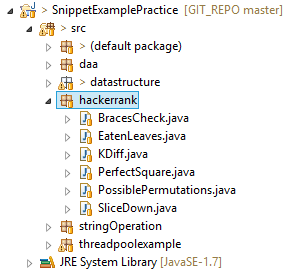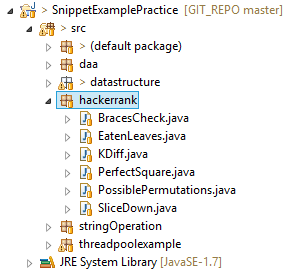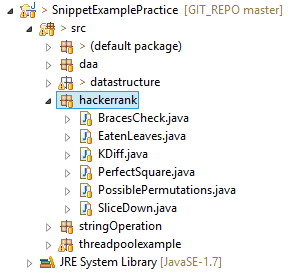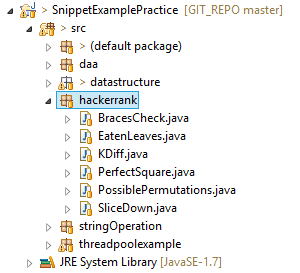# Tag Archives Hacker Rank coding challenge

So, with the use of hash set, it will be achieved in O(n) where n is the total number of elements in array.

Approach 1.Here the first improvement can be achieved, if you look at the caterpillar array. Here you have [2,4,5]. The easiest catch is the discard those caterpillars which are divisible by other. So which will make our caterpillar array [2,4]. It is because the leaves that are eaten by 4 can also be eaten by 2. So we do not need to loop over all the caterpillars. This way we can save many iterations by reducing unnecessary caterpillar.Posted inAll,Interview Questionsand taggedCoding Challenge,Count Perfect Square Java,Count Total Number of Perfect Square,Hacker Rank,Hacker Rank coding challenge,Interview Question,java

Iterate over each braces from start to end and do the below step at each iteration.

Posted inAll,Interview Questionsand taggedCoding Challenge,Hacker Rank,Hacker Rank coding challenge,Interview Question,java,KDiff problem Hacker Rank,unique pairs having difference

Input: X = 1 Y = 2 N = 5 Output: [0, 1, 2, 3, 4] [0, 1, 2, 3, 5] [0, 1, 2, 4, 5] [0, 1, 2, 4, 6] [0, 1, 3, 4, 5] [0, 1, 3, 4, 6] [0, 1, 3, 5, 6] [0, 1, 3, 5, 7] [0, 2, 3, 4, 5] [0, 2, 3, 4, 6] [0, 2, 3, 5, 6] [0, 2, 3, 5, 7] [0, 2, 4, 5, 6] [0, 2, 4, 5, 7] [0, 2, 4, 6, 7] [0, 2, 4, 6, 8]c. If no, then add it to normal hash set

Posted inAll,Interview Questionsand taggedCoding Challenge,Count Duplicate Element,Hacker Rank,Hacker Rank coding challenge,Interview Question,java

Step 2: Iterate over each element in set

import java.util.HashSet; public class CountDuplicates static int countDuplicates(int[] numbers) HashSetInteger set = new HashSetInteger(); HashSetInteger duplicateSet = new HashSetInteger(); for(int i=0;inumbers.length;i++) ntains(numbers[i])) duplicateSet.add(numbers[i]); else set.add(numbers[i]); return duplicateSet.size(); public static void main(String p[]) int a[]=20,20,20,30,40,50,60,90,80,90,100; System.out.println(Total Number having duplicate Count:+countDuplicates(a));

Calculate Fibonacci number in java using Recursion and Multi Threading

To print all the possible permutations in sorted order, in given form, starting from zero.

So the basic approach would be to use stack to verify the correct order of braces.

Verify preorder sequence of Binary Search Tree (BST) Interview Question Hacker Rank

You can also clone the repository from GIT using the link below:

You can also clone the repository from GIT using the link below:

Posted inAll,Interview Questionsand taggedCoding Challenge,Hacker Rank,Hacker Rank coding challenge,Interview Question,java,Slice Down

Count Duplicate Pairs of Integer in a given array (Hacker Rank Interview Questions)And then compute the ceil of the square root of 100 that would be 10.

Total Number of Perfect Squares (Hacker Rank Interview Question)

You can also clone the repository from GIT using the link below:

The worst approach would be to loop over each element and check whether it is an integer.Output: Total number of duplicate numbers present in the given array

b. If yes, then add it to duplicate setHere the naïve approach would be to loop over all the leaves and also loop over all the caterpillars and discard those leaves that are divisible by it. It will have 0 (n2) complexity.

Below is the screen shots of my project directory and the code for the above solution:

2. Do not copy element to another array, just simple store the last index value and decrease it in recursive call.

1 2 5 9    (Subtracted 3 from each elements)

Here in this problem, the input will be an array of integer and also the difference value. So it will ask to out put either the total unique pairs of elements from the array having difference or just total count of that pairs.Again you can find the above code on git repo:

Match the braces and verify weather all the opening braces has the closing braces in right order.

Posted inAll,Interview Questionsand taggedCoding Challenge,Eaten leaves problem,Hacker Rank,Hacker Rank coding challenge,Interview Question,java,The catter pillar problem4             (Subtracted 3 from each elements)

At each iteration, find minimum number and subtract it from element and print non zero elements

Input: Total Leaves N = 10 Caterpillars: 2,4,5,10,12 Output: Reduced Caterpillars:[2, 5] Survived Leaves Count:4 Input: Total Leaves N = 20 Caterpillars: 2,3,4,5 Output: Reduced Caterpillars:[2, 3, 5] Survived Leaves Count:6 Input: Total Leaves N = 20 Caterpillars: 2,4,5,10,12 Output: Reduced Caterpillars:[2, 5] Survived Leaves Count:8

Step 2.  When you find closing bracket: Do pop and check whether closing and opening bracket match. If yes, then continue, if not then break and return NO.

3 7          (Subtracted 1 from each elements)Output: print each iteration by subtracting the smallest elements till array becomes empty

Posted inAll,Interview Questionsand taggedCoding Challenge,Hacker Rank,Hacker Rank coding challenge,Interview Question,java,Java Stack questions,Parentheses parser,Parenthesis check

The output of above code is as below:

Step 3: output the size of duplicate hash set

The best approach would be to determine the floor of root of first integer and ceil of root of last integer.we have leaves [ 1, 2, 3, 4, 5, 6, 7, 8, 9, 10]There are 2 place where you can improve on memory as well as the time complexity (less iteration).

Parentheses / Brackets Check (Hacker Rank Interview Questions)

Caterpillar 2 can eat: 2, 4, 6, 8, 10Now the question will be like, how many leaves will be left if it will be eaten in a way where (Leaf % catterpillar == 0).

import java.util.Scanner; public class PerfectSquare public static void main(String args[]) Scanner in = new ); System.out.println(Please Enter Your Lower Limit); int low = in.nextInt(); System.out.println(Please Enter Your Upper Limit); int high = in.nextInt(); in.close(); int smallestNumber = (int)Math.ceil(Math.sqrt(low)); int highestNumber = (int)Math.floor(Math.sqrt(high)); System.out.println(Total Number of Perfect square are:+(highestNumber-smallestNumber+1));

Here, you will have fixed size array with integer elements called catter pillars.  For example: [2,4,5] and you have integer value of totalLeaves. For example 10, so it will have values from 1 to 10.

Step 1: Create a Set of all the elements.

Below is the java implementation and sample output.Below is the code in java for the above problem.

Print all the possible permutations by adding X and Y (Hacker Rank Interview Question)

Find unique pairs with k difference (KDiff) (Hacker Rank Interview Question)

import java.util.ArrayList; public class EatenLeaves public static void main(String args[]) int inputArray[] = 2,4,5,10,12; System.out.println(Survived Leaves Count:+returnCountLeaves(10,inputArray)); static int returnCountLeaves(int N, int[] A ) ArrayListInteger reduceArrayList = new ArrayListInteger(); reduceArrayList.add(A); boolean reduceFlag = false; for(int i=1;iA.length;i++) reduceFlag = false; for(int j=0;jreduceArrayList.size();j++) if(A[i]%reduceArrayList.get(j)==0) reduceFlag = true; break; if(!reduceFlag) reduceArrayList.add(A[i]); System.out.println(Reduced Caterpillars:+reduceArrayList); int survivedLeaves = 0; for(int m = 1;m=N;m++) for(int j=0;jreduceArrayList.size();j++) if(m%reduceArrayList.get(j) == 0) survivedLeaves++; break; return N-survivedLeaves;

a. Check whether element is in the Hash set

import java.util.Arrays; import java.util.List; import java.util.Scanner; import java.util.Stack; public class BracesCheck public static void main(String args[]) Scanner in = new ); System.out.println(Please Enter Your String); String inputString = in.nextLine(); System.out.println(checkBraces(inputString)); in.close(); static String checkBraces(String value) StackCharacter specialCharStack = new StackCharacter(); String response = Fail; char tempChar; Character[] openingBraces = [,(,; Character[] closingBraces = ],),; ListCharacter openingBracesList = Arrays.asList(openingBraces); ListCharacter closingBracesList = Arrays.asList(closingBraces); if(value == null) return response; else if(value.length()==0) response = Pass; else for(int i=0; i value.length(); i++) tempChar = value.charAt(i); ntains(tempChar)) specialCharStack.push(tempChar); else if(closingBracesList.contains(tempChar)) if(!specialCharStack.isEmpty()) if(tempChar==) && ( != specialCharStack.pop()) return response; else if(tempChar== && !=specialCharStack.pop()) return response; else if(tempChar==] && [ != specialCharStack.pop()) return response; else return response; else return response; if( specialCharStack.isEmpty()) response = Pass; return response; else return response;

So first compute the floor of the square root of 10 that would be 3.

Input: String of braces only:[](()()]

At each recursion step, we will call the method by passing values of X or Y in order. The end of the recursion would be the length of array reaching the value of N.

Problem: Finding the number of perfect squares between two integer range.

The output of above code is as below:

import java.util.ArrayList; public class PossiblePermutations int x=1; int y=2; int permutSize = 5; int length; public static void main(String args[]) PossiblePermutations pd = new PossiblePermutations(); ArrayListInteger al = new ArrayListInteger(); al.add(0); pd.printAllPermutations(al); public void printAllPermutations(ArrayListInteger a) ArrayListInteger a1 = new ArrayListInteger(a); if(a.size() == permutSize) System.out.println(a); else a.add(a.get(a.size()-1) + x); printAllPermutations(a); a1.add(a1.get(a1.size()-1) + y); printAllPermutations(a1); public void printArray(int a[]) for(int i=0;ia.length;i++) System.out.print(a[i]+ ); System.out.println();Posted inAll,Interview Questionsand taggedAll permutations java,Coding Challenge,Hacker Rank,Hacker Rank coding challenge,Interview Question,java,Print all permutations

When Binary Search Tree can perform worst?

X and Y are integer values, where X Y10 3 = 7 will be the output. It will be O(1) .

To solve this problem, we can use recursion by passing the value of X and Y with the current state array.

import java.util.HashSet; import java.util.Iterator; public class KDiff public static void main(String args[]) int pairCount = 0; Integer elements[] = 1,3,4,5,8,8; int kDiff = 2; if(elements.length1 && kDiff=1 && elements.length == elements.length) HashSetInteger hSet = new HashSetInteger(); for(int i=0;ielements.length;i++) int inputElement = elements[i]; ntains(inputElement)) hSet.add(inputElement); IteratorInteger itSet = hSet.iterator(); while (itSet.hasNext()) Integer value = itSet.next(); Integer subtractValue = value+kDiff; if(hSet.contains(subtractValue)) System.out.println(Pair Found:+value+ & +subtractValue); pairCount++; else pairCount=0; System.out.println(Total Pairs:+pairCount);

Step 2: Loop over each element in the given array

Output: total number of perfect squares including this number – int count

1. Identify minimum value while subtracting the minimum value

Step 1.  When you find opening bracket: Do Push.Below code is implementation of above approach.

Output: Total number of unique pairs having difference provided in the input.

Below is the Screenshots of the project structure followed by the source code:Even or Odd String Interview Question Hacker Rank

public class SliceDown public static void main(String args[]) SliceDown sd = new SliceDown(); int a[] = 15,12,10,5,3; printArray(a, a.length); int smallestIndex = findSmallest(a); sd.sliceDown(a,a.length,smallestIndex); /* * recursively call this method by passing the index value of * min number and the range that needs to be sliced down */ public void sliceDown(int a[],int range, int smallestIndex) int new_range = 0; int new_smallestIndex = -1; int smallNumber = a[smallestIndex]; for(int i=0;irange;i++) // To skip the number which is going to be slice down if(a[i]!=smallNumber) a[new_range] = a[i] – smallNumber; //To initialize & find the new minimum number for next iteration if(new_smallestIndex0) new_smallestIndex = new_range; else if(a[new_range] a[new_smallestIndex]) new_smallestIndex = new_range; new_range++; printArray(a,new_range); if(new_range1) sliceDown(a, new_range,new_smallestIndex); //This method will be called only once for the first iteration private static int findSmallest(int a[]) int smallestIndex = 0; for(int i=1;ia.length;i++) if(a[smallestIndex]a[i]) smallestIndex = i; return smallestIndex; public static void printArray(int a[],int range) for(int i=0;irange;i++) System.out.print(a[i]+ ); System.out.println();

The caterpillar problem (Hacker Rank Interview Questions)

Input: String of braces only:[]()[()()]

1 4 8       (Subtracted 1 from each elements)

The use of Hash set can be done in order to solve this by traversing each element and adding it into the set, later on they are checked for repetitive addition (i.e. we make sure that the numbers were not included already!)

Input: first integer and last integer –  int startInteger, int endInteger

Step 1: Create 2 hash sets. 1 is for all the elements and the other is for the duplicate elements.

For the above problem, naive approach is to find the minimum in each iteration, subtracting it and copy it in new memory.

Check whether number (element value + diff) is in hashset.

Input: Integer array with 0 or more repeated values.